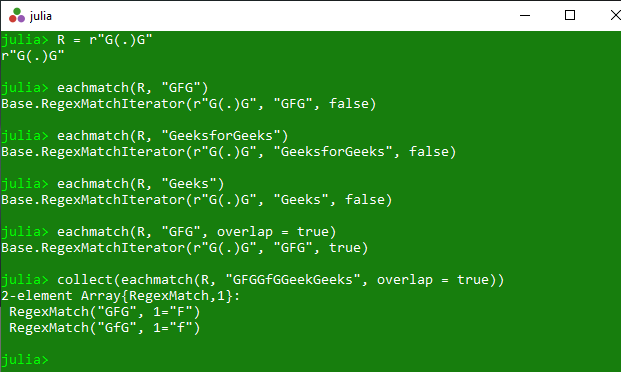Related Articles
Matching of regular expression in string in Julia – match() and eachmatch() Methods
• Last Updated : 20 Apr, 2020

The `match()` is an inbuilt function in julia which is used to search for the first match of the given regular expression r in the specified string s and then returns a RegexMatch object containing the match or nothing if the match failed.

Syntax:
match(r::Regex, s::AbstractString, idx::Integer)

Parameters:

• r::Regex: Specified regular expression.
• s::AbstractString: Specified string.
• idx::Integer: It specifies the point from which the searching get started.

Returns: It returns a RegexMatch object containing the match or nothing if the match failed.

Example:

 `# Julia program to illustrate  ` `# the use of match() method ` `  `  `# Getting a RegexMatch object containing ` `# the match or nothing if the match failed.  ` `R ``=` `r``"G(.)G"` `println(match(R, ``"GFG"``)) ` `println(match(R, ``"Geeks"``)) ` `println(match(R, ``"gfgGfG"``)) ` `println(match(R, ``"GFG"``, ``1``)) ` `println(match(R, ``"GFG"``, ``2``)) `

Output:

```RegexMatch("GFG", 1="F")
nothing
RegexMatch("GfG", 1="f")
RegexMatch("GFG", 1="F")
nothing
```

#### eachmatch()

The `eachmatch()` is an inbuilt function in julia which is used to search for all the matches of the given regular expression r in the specified string s and then returns a iterator over the matches. If overlap is true, the matching sequences are allowed to overlap indices in the original string, otherwise they must be from distinct character ranges.

Syntax:
eachmatch(r::Regex, s::AbstractString; overlap::Bool)

Parameters:

• r::Regex: Specified regular expression.
• s::AbstractString: Specified string.
• overlap::Bool: Specified overlap boolean value.

Returns: It returns a iterator over the matches.

Example:

 `# Julia program to illustrate  ` `# the use of eachmatch() method ` `  `  `# Getting a iterator over the matches ` `R ``=` `r``"G(.)G"` `println(eachmatch(R, ``"GFG"``)) ` `println(eachmatch(R, ``"GeeksforGeeks"``)) ` `println(eachmatch(R, ``"Geeks"``)) ` `println(eachmatch(R, ``"GFG"``, overlap ``=` `true)) ` `println(collect(eachmatch(R, ``"GFGGfGGeekGeeks"``, overlap ``=` `true))) `

Output:My Personal Notes arrow_drop_up
Recommended Articles
Page :